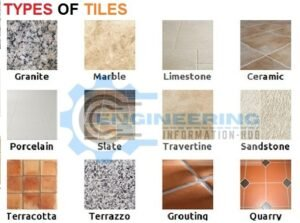# How To Calculate The Cement and Sand For Tiles Fixing 50ft²

In this article, I explain how to calculate the cement in tiles fixing on 50sq.ft area and also explain the benefit of tiles purpose of tiles fixing how much use the thickness of cement using in floor tiles and wall tiles.### what is the purpose of tiles?The basic purpose of tile is to cover the floor and wall and easy to fix it. The different types are used in home and all construction work and also use in road work footpath park area etc. some area are using ceramic tiles marble tiles glass tile cement tiles Gary nite tile limestone tile etc. all tiles are used different purpose.

### How To Estimate The Concrete of Volume For The Staircase

Work for wall

Tile fixing for wall the thickness of cement up to 1inch=1/12=0.083ft

Work for floor

Tile fixing for floor the thickness of cement up to 1.5inch=1.5/12=0.125ft

Now we have the area of room or hall =50ft²

Now we find Work for floor

we find the volume of room or hall

volume of wet mortar =area of room or hall × thickness of cement

Volume of wet mortar= 50×0.125=6.25ft³

Now we convert volume wet to dry

We know the wet volume of any mortar if we convert to dry volume this will be increased by 33%. Multiply the wet volume to 1.33 of the cofactor of dry volume

Dry volume of Mortar= 6.25×1.33=8.312ft³

#### How To Calculate The Cement For Tiles Fixing 50ft²

we use the cement ratio of mortar using in tiles 1:2

volume of cement = cement ratio/sum of ratio × dry volume of mortar

volume of cement = 1/3×8.312=2.77ft³

we know the 20% extra cement used for adhesive.

so total volume of cement

2.77/100×20=0.554ft³

Total volume of cement =2.77+0.554=3.324ft³

### Different Types Of Stone Masonry Used in Construction

volume of one bag cement =1.25ft³

The bag of cement=volume of dry cement/volume of one bag

The bag of cement=3.324/1.25=2.659 bag= 3bags

The same step is used to find the volume for the wall but use the thickness of cement 01 inches.

Now calculate the volume of sand

volume of sand=ratio of sand/sum of ratio×dry volume of mortar

volume of sand=2/3×8.31=5.54ft³